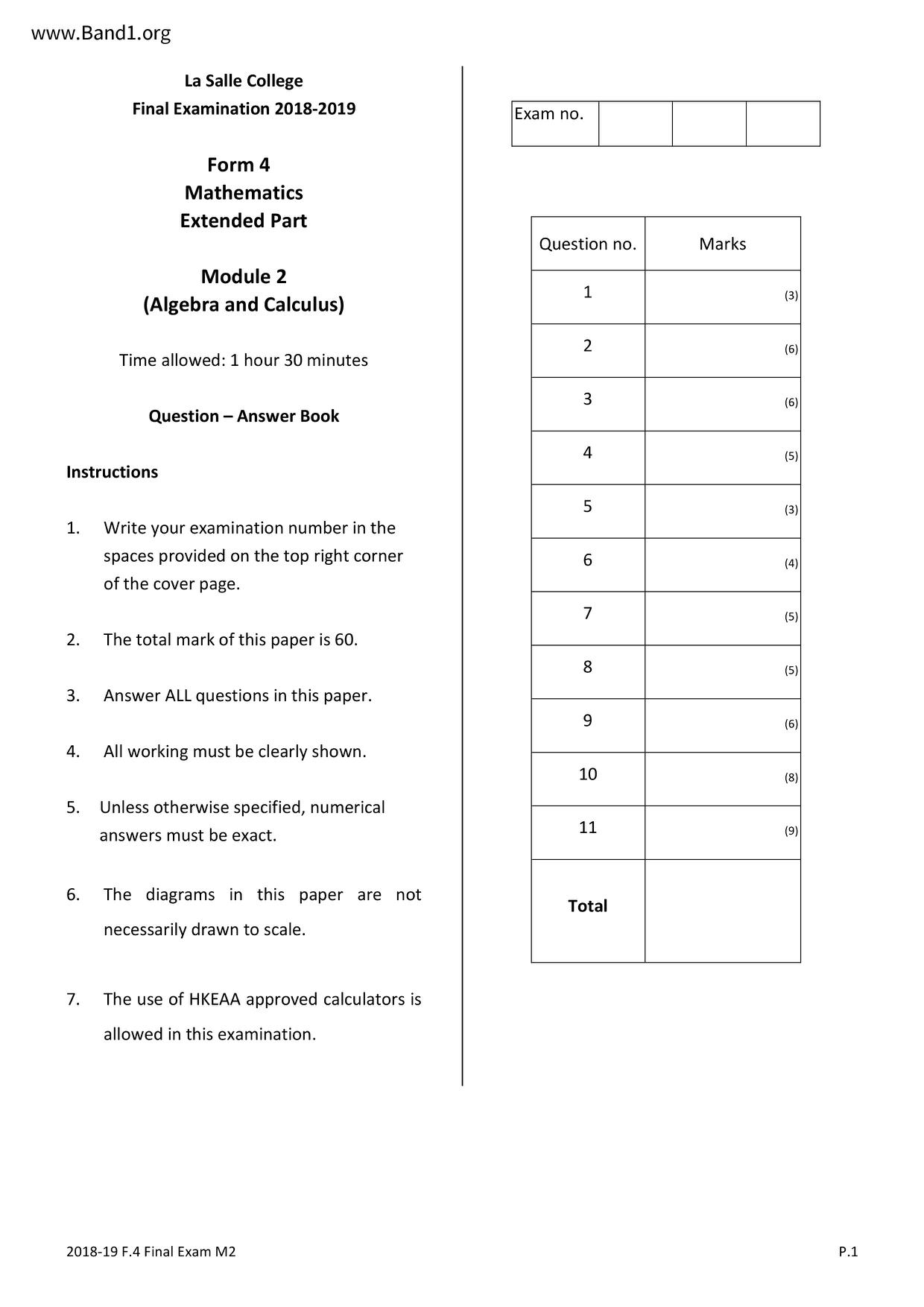# 中四 數學試卷 (F4 Maths Past Paper)

6629

pdf

16

maths _FINAL_M2_1819

▼ 圖片只作預覽, 如欲下載整份卷, 請按「免費成為會員」 ▼▲ 圖片只作預覽, 如欲下載整份卷, 請按「免費成為會員」 ▲

## 中四數學試卷 PDF 下載

Instructions
La Salle College
Final Examination 2018-2019
Mathematics
Extended Part
(Algebra and Calculus)
Time allowed: 1 hour 30 minutes
Write your examination number in the
spaces provided on the top right corner
of the cover page.
The total mark of this paper is 60.
Answer ALL questions in this paper.
5. Unless otherwise specified, numerical
All working must be clearly shown.
The diagrams in this paper are not
necessarily drawn to scale.
The use of HKEAA approved calculators is
allowed in this examination.
2018-19 F.4 Final Exam M2
Question no.
(a) Given sin(a+0) = k sin(α-0), where k# 1. Find the value of
Hence, or otherwise, solve the equation sin 2x+
2018-19 F.4 Final Exam M2
in terms of k.
for 0≤x≤ 2π.
2018-19 F.4 Final Exam M2
(a) Using mathematical induction, prove that sin 0Σ cos(2k – 1) 0
1) 0 = sin 2n0 for all positive
integers n.
Using (a), evaluate
2018-19 F.4 Final Exam M2
lim cos(2k-1)0.
2018-19 F.4 Final Exam M2
In the figure, AABC is a triangle with ZBAC = 0.
P is a point on AB such that PA = PB = PC = r.
R and Q are points on AC and BC respectively such that ZQPC = ZRPC = 0 .
Show that PR =
Find ZPCQ in terms of 0. Hence show that PQ =
Show that the area of APQR
2018-19 F.4 Final Exam M2
cos (0 - 0)*
sin 20 + sin 20
r² sin 20 (1
2018-19 F.4 Final Exam M2
2018-19 F.4 Final Exam M2
~~End of Paper~~
sin(A+B) = sinAcosB + cosAsinB
cos(A+B) = cosAcosB + sinAsinB
tan A + tan B
1+ tan A tan B
tan (A+B) =-
sinA+sinB = 2sin
sinA-sinB = 2cos
2018-19 F.4 Final Exam M2
Formulas for Reference
2sinAcosB = sin(A+B)+sin(A-B)
2cosAcosB = cos(A+B)+cos(A-B)
2sinAsinB = cos(A-B) — cos(A+B)
cosA+cosB = 2cos
cosA-cosB = -2sin
Find the value of tan165°. Express the answer in the form p + q
+ q√√3, where р and q are integers.
of the following functions.
X = y² + y + 1
(c) y (x − 2) = (y + 1)²
2018-19 F.4 Final Exam M2
lim (x+√√x² +7x).
lim (x+1) In
2018-19 F.4 Final Exam M2
In the expansion of (1 + ax²)", the coefficients of x² and x4 are -32 and 448 respectively.
Find the values of a and n.
Hence find the coefficient of x² in the expansion (1 + ax ²)¹ (1 − x)(1 + x).
2018-19 F.4 Final Exam M2
Solve e5x10e4x + 40e³x - 80e²x + 80e* +211 = 0.
2018-19 F.4 Final Exam M2
d(et cos x)
from first principles.
2018-19 F.4 Final Exam M2
Hence, or otherwise, solve the equation 1 - cos x = sin x
(a) Prove that tan
2018-19 F.4 Final Exam M2
for 0≤x≤ 2π.
Expand e-2x in ascending powers of x as far as the term in x².
(b) Expand (1 + 3x)e-2x in ascending powers of x as far as the term in x².
(c) Using (b), find an approximation to 0.2 as a fraction in its simplest form.
2018-19 F.4 Final Exam M2